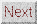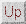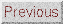# waynehu

## Professor, Department of Astronomy and Astrophysics University of Chicago

Group Contact CV SnapShots

## Gravitational Forcing

We saw above that fluctuations in a scalar field during inflation get turned into temperature fluctuations via the intermediary of gravity. Gravity affects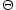in more ways than this. The Newtonian potential and spatial curvature alter the acoustic oscillations by providing a gravitational force on the oscillator. The Euler equation (8) gains a term on the rhs due to the gradient of the potential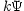. The main effect of gravity then is to make the oscillations a competition between pressure gradients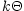and potential gradientswith an equilibrium when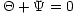.

Gravity also changes the continuity equation. Since the Newtonian curvature is essentially a perturbation to the scale factor, changes in its value also generate temperature perturbations by analogy to the cosmological redshift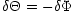and so the continuity equation (7) gains a contribution of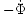on the rhs.

These two effects bring the oscillator equation (9) to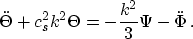(14)

In a flat universe and in the absence of pressure,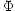and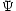are constant. Also, in the absence of baryons,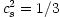so the new oscillator equation is identical to Equation (9) withreplaced by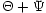. The solution in the matter dominated epoch is then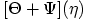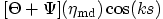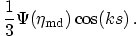(15)

where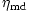represents the start of the matter dominated epoch (see Figure 1a). We have used the matter dominated initial conditions'' forgiven in the previous section assuming large scales,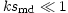.

The results from the idealization of §3.1 carry through with a few exceptions. Even without an initial temperature fluctuation to displace the oscillator, acoustic oscillations would arise by the infall and compression of the fluid into gravitational potential wells. Since it is the effective temperaturethat oscillates, they occur even if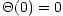. The quantitycan be thought of as an effective temperature in another way: after recombination, photons must climb out of the potential well to the observer and thus suffer a gravitational redshift of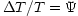. The effective temperature fluctuation is therefore also the observed temperature fluctuation. We now see that the large scale limit of Equation (15) recovers the famous Sachs-Wolfe result that the observed temperature perturbation is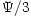and overdense regions correspond to cold spots on the sky [Sachs & Wolfe, 1967]. When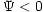, althoughis positive, the effective temperatureis negative. The plasma begins effectively rarefied in gravitational potential wells. As gravity compresses the fluid and pressure resists, rarefaction becomes compression and rarefaction again. The first peak corresponds to the mode that is caught in its first compression by recombination. The second peak at roughly half the wavelength corresponds to the mode that went through a full cycle of compression and rarefaction by recombination. We will use this language of the compression and rarefaction phase inside initially overdense regions but one should bear in mind that there are an equal number of initially underdense regions with the opposite phase.